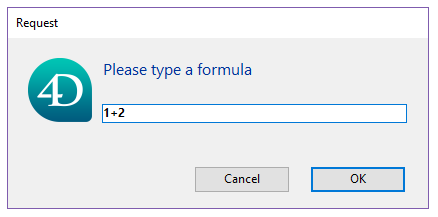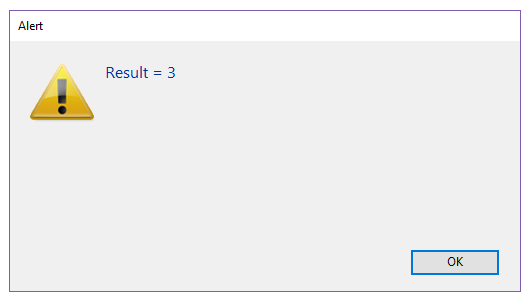Version: v19

# Function

A `4D.Function` object contains a piece of code that can be executed from an object, either using the `()` operator, or using the `apply()` and `call()` functions. 4D proposes three kinds of `Function` objects:

• native functions, i.e. built-in functions from various 4D classes such as `collection.sort()` or `file.copyTo()`.
• user functions, created in user classes using the Function keyword.
• formula functions, i.e. functions that can execute any 4D formula.

### Formula Objects​

The Formula and Formula from string commands allow you to create `4D.Function` objects to execute any 4D expression or code expressed as text.

Formula objects can be encapsulated in object properties:

`` var \$f : 4D.Function \$f:=New object \$f.message:=Formula(ALERT("Hello world"))``

This property is an "object function", i.e. a function which is bound to its parent object. To execute a function stored in an object property, use the () operator after the property name, such as:

`` \$f.message() //displays "Hello world"``

Syntax with brackets is also supported:

`` \$f["message"]() //displays "Hello world"``

Note that, even if it does not have parameters (see below), an object function to be executed must be called with ( ) parenthesis. Calling only the object property will return a new reference to the formula (and will not execute it):

`` \$o:=\$f.message //returns the formula object in \$o``

You can also execute a function using the `apply()` and `call()` functions:

`` \$f.message.apply() //displays "Hello world"``

#### Passing parameters​

You can pass parameters to your formulas using the sequential parameter syntax based upon \$1, \$2...\$n. For example, you can write:

`` var \$f : Object \$f:=New object \$f.message:=Formula(ALERT("Hello "+\$1)) \$f.message("John") //displays "Hello John"``

Or using the .call() function:

`` var \$f : Object \$f:=Formula(\$1+" "+\$2) \$text:=\$f.call(Null;"Hello";"World") //returns "Hello World" \$text:=\$f.call(Null;"Welcome to";String(Year of(Current date))) //returns "Welcome to 2019" (for example)``

#### Parameters to a single method​

For more convenience, when the formula is made of a single project method, parameters can be omitted in the formula object initialization. They can just be passed when the formula is called. For example:

`` var \$f : 4D.Function \$f:=Formula(myMethod)  //Writing Formula(myMethod(\$1;\$2)) is not necessary \$text:=\$f.call(Null;"Hello";"World") //returns "Hello World" \$text:=\$f.call() //returns "How are you?"  //myMethod #DECLARE (\$param1 : Text; \$param2 : Text)->\$return : Text If(Count parameters=2)    \$return:=\$param1+" "+\$param2 Else    \$return:="How are you?" End if``

Parameters are received within the method, in the order they are specified in the call.

### Summary​

.apply() : any
.apply( thisObj : Object { ; formulaParams : Collection } ) : any
executes the `formula` object to which it is applied and returns the resulting value
.call() : any
.call( thisObj : Object { ; ...params : any } ) : any
executes the `formula` object to which it is applied and returns the resulting value
.source : Text     contains the source expression of the `formula` as text

## Formula​

History
VersionChanges
v17 R6Renamed (New formula -> Formula)

Formula ( formulaExp : Expression ) : 4D.Function

ParameterTypeDescription
formulaExpExpression->Formula to be returned as object
Result4D.Function<-Native function encapsulating the formula

#### Description​

The `Formula` command creates a `4D Function` object based upon the formulaExp expression. formulaExp can be as simple as a single value or complex, such as a project method with parameters.

Having a formula as an object allows it to be passed as a parameter (calculated attribute) to commands or methods or to be executed from various components without needing to declare them as "shared by components and host database". When called, the formula object is evaluated within the context of the database or component that created it.

The returned formula can be called with:

`` var \$f : 4D.Function \$f:=Formula(1+2) \$o:=New object("myFormula";\$f)  //three different ways to call the formula \$f.call(\$o) //returns 3 \$f.apply(\$o) //returns 3 \$o.myFormula() //returns 3``

You can pass parameters to the `Formula`, as seen below in example 4.

You can specify the object on which the formula is executed, as seen in example 5. The properties of the object can then be accessed via the `This` command.

If formulaExp uses local variables, their values are copied and stored in the returned formula object when it is created. When executed, the formula uses these copied values rather than the current value of the local variables. Note that using arrays as local variables is not supported.

The object created by `Formula` can be saved, for example, in a database field or in a blob document.

#### Example 1​

A simple formula:

`` var \$f : 4D.Function \$f:=Formula(1+2) var \$o : Object \$o:=New object("f";\$f) \$result:=\$o.f() // returns 3``

#### Example 2​

A formula using local variables:

`` \$value:=10 \$o:=New object("f";Formula(\$value)) \$value:=20 \$result:=\$o.f() // returns 10``

#### Example 3​

A simple formula using parameters:

`` \$o:=New object("f";Formula(\$1+\$2)) \$result:=\$o.f(10;20) //returns 30``

#### Example 4​

A formula using a project method with parameters:

`` \$o:=New object("f";Formula(myMethod)) \$result:=\$o.f("param1";"param2") // equivalent to \$result:=myMethod("param1";"param2")``

#### Example 5​

Using `This`:

`` \$o:=New object("fullName";Formula(This.firstName+" "+This.lastName)) \$o.firstName:="John" \$o.lastName:="Smith" \$result:=\$o.fullName() //returns "John Smith"``

#### Example 6​

Calling a formula using object notation:

`` var \$feta; \$robot : Object var \$calc : 4D.Function \$robot:=New object("name";"Robot";"price";543;"quantity";2) \$feta:=New object("name";"Feta";"price";12.5;"quantity";5) \$calc:=Formula(This.total:=This.price*This.quantity)  //sets the formula to object properties \$feta.calc:=\$calc \$robot.calc:=\$calc  //call the formula \$feta.calc() // \$feta={name:Feta,price:12.5,quantity:5,total:62.5,calc:"[object Formula]"} \$robot.calc() // \$robot={name:Robot,price:543,quantity:2,total:1086,calc:"[object Formula]"}``

## Formula from string​

History
VersionChanges
v17 R6Renamed New formula from string -> Formula from string

Formula from string( formulaString : Text ) : 4D.Function

ParameterTypeDescription
formulaStringText->Text formula to be returned as object
Result4D.Function<-Native object encapsulating the formula

#### Description​

The `Formula from string` command creates a 4D.Function object based upon the formulaString. formulaString can be as simple as a single value or complex, such as a project method with parameters.

This command is similar to `Formula`, except that it handles a text-based formula. In most cases, it is recommended to use the `Formula` command. `Formula from string` should only be used when the original formula was expressed as text (e.g., stored externally in a JSON file). In this context, using syntax with tokens is highly advised.

Because local variable contents can not be accessed by name in compiled mode, they can not be used in formulaString. An attempt to access a local variable with `Formula from string` will result in an error (-10737).

#### Example​

The following code will create a dialog accepting a formula in text format:

`` var \$textFormula : Text var \$f : 4D.Function \$textFormula:=Request("Please type a formula") If(ok=1)    \$f:=Formula from string(\$textFormula)    ALERT("Result = "+String(\$f.call())) End if``...and execute the formula:## .apply()​

History
VersionChanges

.apply() : any
.apply( thisObj : Object { ; formulaParams : Collection } ) : any

ParameterTypeDescription
thisObjObject->Object to be returned by the This command in the formula
formulaParamsCollection->Collection of values to be passed as \$1...\$n when `formula` is executed
Resultany<-Value from formula execution

#### Description​

The `.apply()` function executes the `formula` object to which it is applied and returns the resulting value. The formula object can be created using the `Formula` or `Formula from string` commands.

In the thisObj parameter, you can pass a reference to the object to be used as `This` within the formula.

You can also pass a collection to be used as \$1...\$n parameters in the formula using the optional formulaParams parameter.

Note that `.apply()` is similar to `.call()` except that parameters are passed as a collection. This can be useful for passing calculated results.

#### Example 1​

`` var \$f : 4D.Function \$f:=Formula(\$1+\$2+\$3) \$c:=New collection(10;20;30) \$result:=\$f.apply(Null;\$c) // returns 60``

#### Example 2​

`` var \$calc : 4D.Function var \$feta; \$robot : Object \$robot:=New object("name";"Robot";"price";543;"quantity";2) \$feta:=New object("name";"Feta";"price";12.5;"quantity";5) \$calc:=Formula(This.total:=This.price*This.quantity) \$calc.apply(\$feta) // \$feta={name:Feta,price:12.5,quantity:5,total:62.5} \$calc.apply(\$robot) // \$robot={name:Robot,price:543,quantity:2,total:1086}``

## .call()​

History
VersionChanges

.call() : any
.call( thisObj : Object { ; ...params : any } ) : any

ParameterTypeDescription
thisObjObject->Object to be returned by the This command in the formula
paramsany->Value(s) to be passed as \$1...\$n when formula is executed
Resultany<-Value from formula execution

#### Description​

The `.call()` function executes the `formula` object to which it is applied and returns the resulting value. The formula object can be created using the `Formula` or `Formula from string` commands.

In the thisObj parameter, you can pass a reference to the object to be used as `This` within the formula.

You can also pass values to be used as \$1...\$n parameters in the formula using the optional params parameter(s).

Note that `.call()` is similar to `.apply()` except that parameters are passed directly.

#### Example 1​

`` var \$f : 4D.Function \$f:=Formula(Uppercase(\$1)) \$result:=\$f.call(Null;"hello") // returns "HELLO"``

#### Example 2​

`` \$o:=New object("value";50) \$f:=Formula(This.value*2) \$result:=\$f.call(\$o) // returns 100``

## .source​

History
VersionChanges
The `.source` property contains the source expression of the `formula` as text.
`` var \$of : 4D.Function var \$tf : Text \$of:=Formula(String(Current time;HH MM AM PM)) \$tf:=\$of.source //"String(Current time;HH MM AM PM)"``